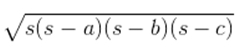Guru

# A kite in the shape of a square with a diagonal 32 cm and an isosceles triangle of base 8 cm and sides 6 cm each is to be made of three different shades as shown in Fig. 12.17. How much paper of each shade has been used in it? Q.7

• 0

Yesterday i was doing the question from class 9th ncert book of math of Heron’s Formula chapter of exercise 12.2    What is the easiest way for solving it because i was not able to do this question please help me for solving this question no. 7 A kite in the shape of a square with a diagonal 32 cm and an isosceles triangle of base 8 cm and sides 6 cm each is to be made of three different shades as shown in Fig. 12.17. How much paper of each shade has been used in it?

Share

1. As the kite is in the shape of a square, its area will be

A = (½)×(diagonal)2

Area of the kite = (½)×32×32 = 512 cm2.

The area of shade I = Area of shade II

512/2 cm2 = 256 cm2

So, the total area of the paper that is required in each shade = 256 cm2

For the triangle section (III),

The sides are given as 6 cm, 6 cm and 8 cm

Now, the semi perimeter of this isosceles triangle = (6+6+8)/2 cm = 10 cm

By using Heron’s formula, the area of the III triangular piece will be

== √[10(10-6)(10-6)(10-8)] cm2

= √(10×4 ×4×2) cm2

= 8√5 cm2 = 17.92 cm2 (approx.)

• 0